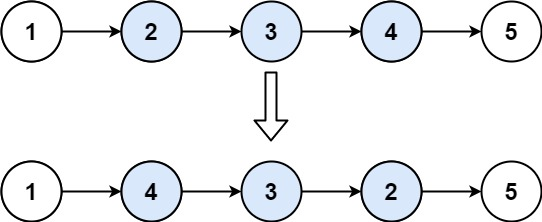304 North Cardinal St.
Dorchester Center, MA 02124

# Reverse Linked List II LeetCode Solution

## Problem – Reverse Linked List II LeetCode Solution

Given the `head` of a singly linked list and two integers `left` and `right` where `left <= right`, reverse the nodes of the list from position `left` to position `right`, and return the reversed list.

Example 1:``````Input: head = [1,2,3,4,5], left = 2, right = 4
Output: [1,4,3,2,5]
``````

Example 2:

``````Input: head = , left = 1, right = 1
Output: 
``````

Constraints:

• The number of nodes in the list is `n`.
• `1 <= n <= 500`
• `-500 <= Node.val <= 500`
• `1 <= left <= right <= n`

Follow up: Could you do it in one pass?

## Reverse Linked List II LeetCode Solution in Java

``````public ListNode reverseBetween(ListNode head, int m, int n) {
ListNode dummy = new ListNode(0); // create a dummy node to mark the head of this list
ListNode pre = dummy; // make a pointer pre as a marker for the node before reversing
for(int i = 0; i<m-1; i++) pre = pre.next;

ListNode start = pre.next; // a pointer to the beginning of a sub-list that will be reversed
ListNode then = start.next; // a pointer to a node that will be reversed

// 1 - 2 -3 - 4 - 5 ; m=2; n =4 ---> pre = 1, start = 2, then = 3
// dummy-> 1 -> 2 -> 3 -> 4 -> 5

for(int i=0; i<n-m; i++)
{
start.next = then.next;
then.next = pre.next;
pre.next = then;
then = start.next;
}

// first reversing : dummy->1 - 3 - 2 - 4 - 5; pre = 1, start = 2, then = 4
// second reversing: dummy->1 - 4 - 3 - 2 - 5; pre = 1, start = 2, then = 5 (finish)

return dummy.next;

}
``````

## Reverse Linked List II LeetCode Solution in C++

``````class Solution {
public:
ListNode* reverseBetween(ListNode* head, int m, int n) {
ListNode *dummy = new ListNode(0), *pre = dummy, *cur;
for (int i = 0; i < m - 1; i++) {
pre = pre -> next;
}
cur = pre -> next;
for (int i = 0; i < n - m; i++) {
ListNode* temp = pre -> next;
pre -> next = cur -> next;
cur -> next = cur -> next -> next;
pre -> next -> next = temp;
}
return dummy -> next;
}
};
``````

## Reverse Linked List II LeetCode Solution in Python

``````class Solution:
# @param m, an integer
# @param n, an integer
# @return a ListNode
if m == n:

dummyNode = ListNode(0)
pre = dummyNode

for i in range(m - 1):
pre = pre.next

# reverse the [m, n] nodes
reverse = None
cur = pre.next
for i in range(n - m + 1):
next = cur.next
cur.next = reverse
reverse = cur
cur = next

pre.next.next = cur
pre.next = reverse

return dummyNode.next
``````
##### Reverse Linked List II LeetCode Solution Review:

In our experience, we suggest you solve this Reverse Linked List II LeetCode Solution and gain some new skills from Professionals completely free and we assure you will be worth it.

If you are stuck anywhere between any coding problem, just visit Queslers to get the Reverse Linked List II LeetCode Solution

Find on Leetcode

##### Conclusion:

I hope this Reverse Linked List II LeetCode Solution would be useful for you to learn something new from this problem. If it helped you then don’t forget to bookmark our site for more Coding Solutions.

This Problem is intended for audiences of all experiences who are interested in learning about Data Science in a business context; there are no prerequisites.

Keep Learning!

More Coding Solutions >>

LeetCode Solutions

Hacker Rank Solutions

CodeChef Solutions